LaTeX 排版过程中有不少（约定俗成的）规则，它们是区别「熟练掌握 LaTeX」与「会使用 LaTeX」的关键因素。

LaTeX 排版过程中有不少（约定俗成的）规则，它们是区别「熟练掌握 LaTeX」与「会使用 LaTeX」的关键因素。这些规则或习惯，有一些不是必须的，做好以后排版会更加美观，有些则是必须的，做不好就是错误的。记录在此，以作备忘！

#### 改变字体属性

\bf\it\rm\sc\sf 不要用了，没有叠加效果、已过时。可以用 \textbf{}\textit{}\textrm{}\textsc{}、、\textsl{}

\textit\emph 不一样，有些语系排版「强调」不是用意大利体表现的。
「斜体」\textsl 和「意大利手写体」\textit 也不一样。

times 和 txfonts 宏包已经过时了，很多 bug，尽量用 mathptm 或 newtxtext，newtxmath 宏包。

#### 特殊数学字体

• \mathcal $\rightarrow$ $\mathcal{A}$ 是欧拉书写体字符；
• \mathbb $\rightarrow$ $\mathbb{R}$ 是空心数学字符；
• \mathscr $\rightarrow$ $\mathscr{A}$ 是花体手写字符，需要 mathrsfs 宏包。

#### 正确输入数学算符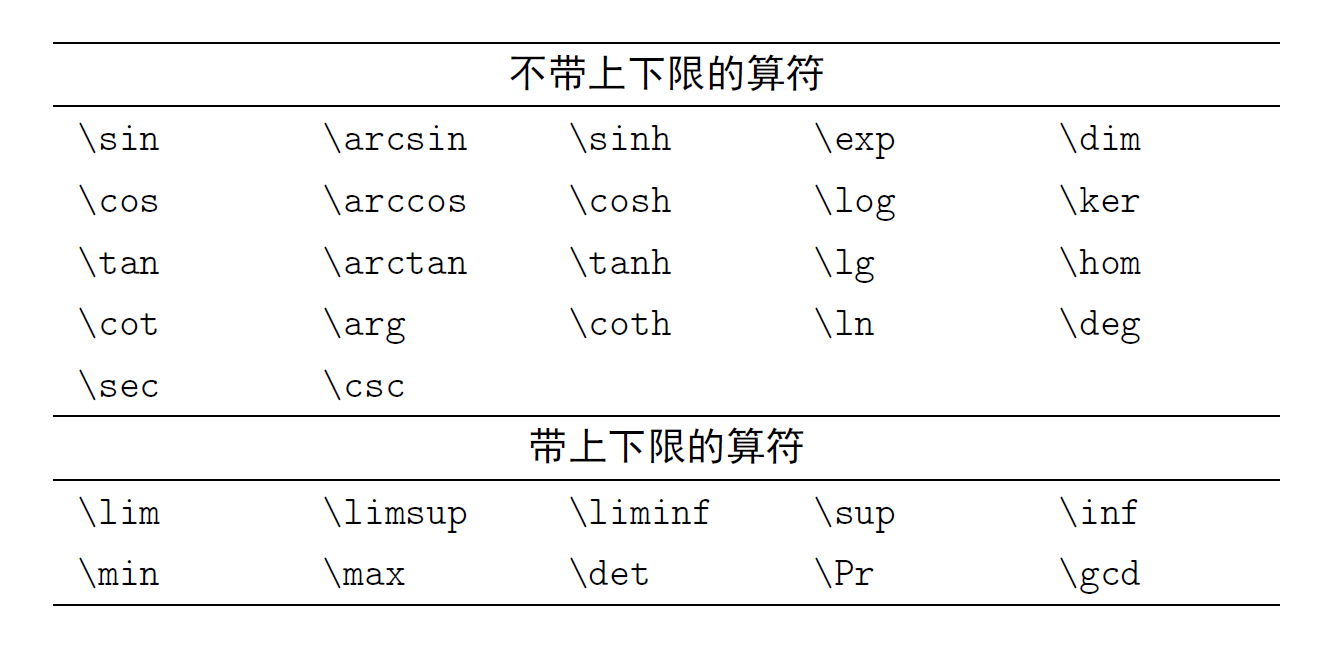% \usepackage{siunitx}
\SI{10}{kg}

$\rightarrow$ 10 kg.

#### 公用编号的多行公式

\begin{equation}
\begin{aligned}
a &= b + c \\
d &= e + f + g \\
h + i &= j + k \\
l + m &= n
\end{aligned}
\end{equation}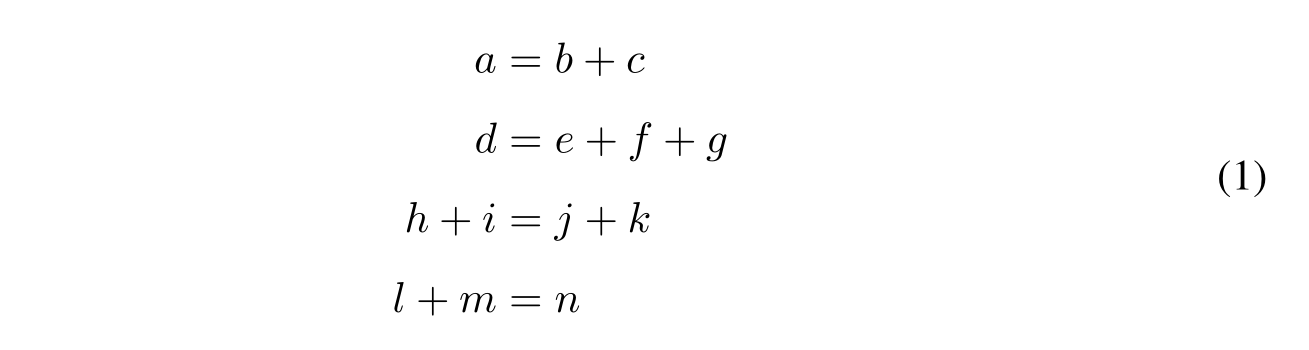\begin{equation}
\begin{aligned}
&a = b + 1 && e = c + f + g + 3 \\
&c = d + e + 2 && c = d + e + 2 \\
&e = c + f + g + 3 && a = b + 1 \\
\end{aligned}
\end{equation}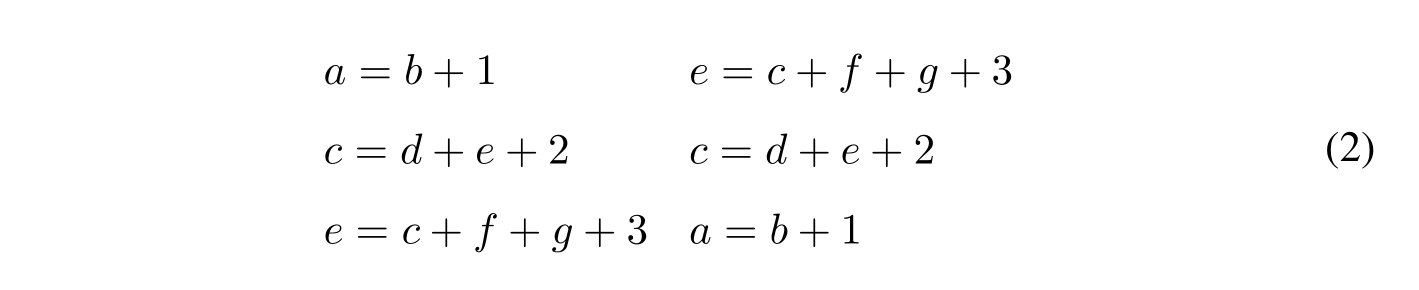aligned 与 equation 环境套用，公式间距是自动调节的，如果有分式，分式也是 displaystyle.

#### [](#微分符号-mathrm-d-x "微分符号 $\mathrm{d}x$")微分符号 $\mathrm{d}x$

• \int f(x) dx $\rightarrow$ $\int f(x) dx$
• \int f(x) \,dx $\rightarrow$ $\int f(x) \,dx$
• \int f(x) \,\mathrm{d}x $\rightarrow$ $\int f(x) \,\mathrm{d}x$

% \usepackage{physics}
$\int f(x) \dd{x}$

$\rightarrow$ $\int f(x) \,\mathrm{d}x$

% \newcommand*{\dif}{\mathop{}\!\mathrm{d}}
$\int f(x) \dif{x}$

$\rightarrow$ $\int f(x) \,\mathrm{d}x$

#### 几种连接符

A specter is haunting Europe --- the specter of Communism.

A specter is haunting Europe—the specter of Communism.

#### 几种转置字符

$\mathbf{A}^\mathrm{T}$
$\mathbf{A}^\top$
$\mathbf{A}^\mathsf{T}$
$\mathbf{A}^\intercal$#### 英文简写的用法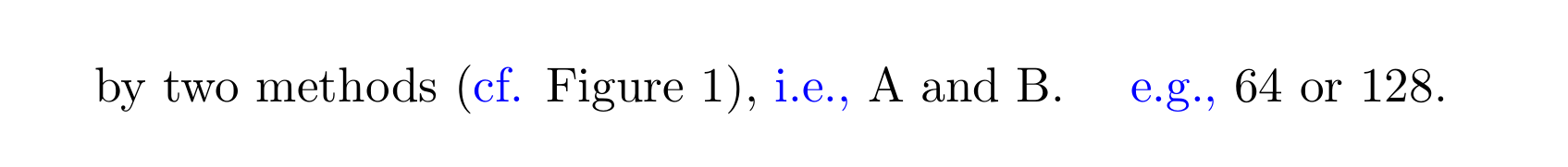#### 公式间距与标点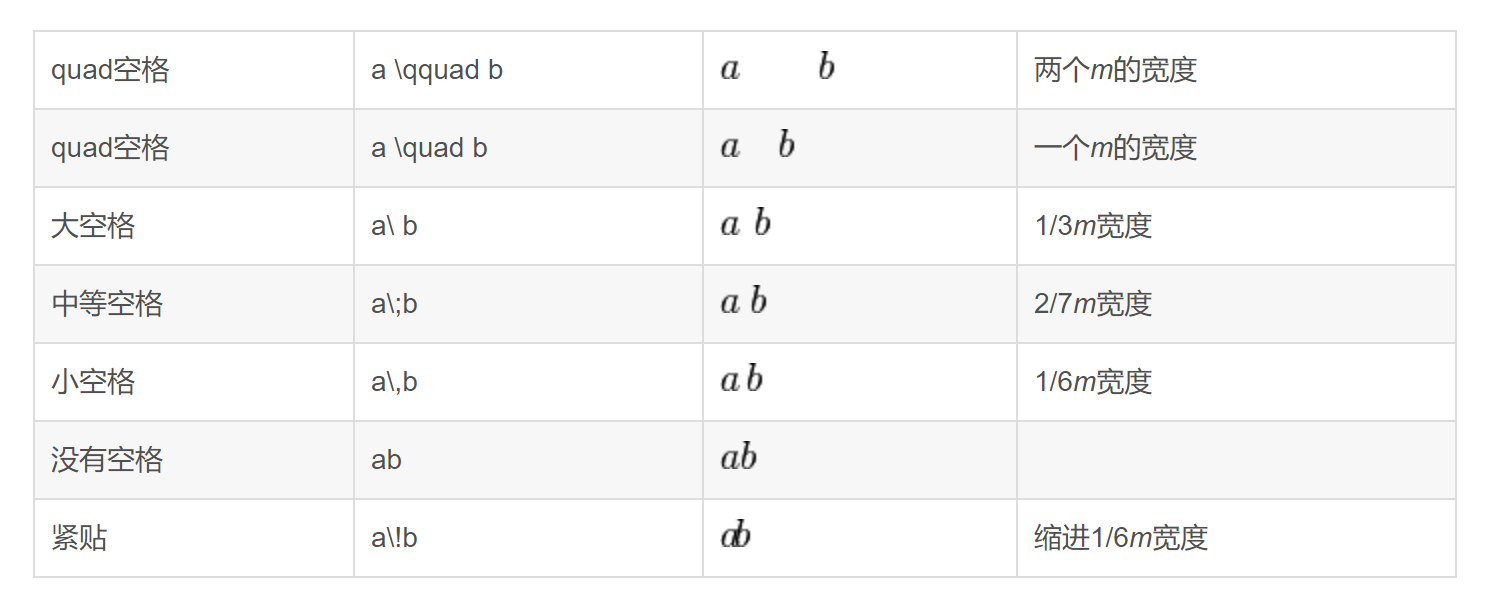\quad1ememm` 代表当前字体下接近字符‘M’的宽度。